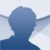# Euler Mathematical Toolbox

2021.12.14

## Euler Mathematical Toolbox is a calculus environment valid both for numerical as well as algebraic computation. Download Euler Mathematical Toolbox for free

Numeric and symbolic computation can be applied to several maths fields: algebra, differential and integral calculus, etc... thus making it easier to complete complex mathematical processes. Maple, MATLAB and Euler Mathematical Toolbox are systems that are well known due to their quality.

Euler Mathematical Toolbox consists of a core focused on numerical calculus developed by R. Grothmann and of the well-known Maxima application for symbolic calculus.

### Main features

• Quickly calculate numerical calculations, represented by means of the IEEE-754 Standard for Floating-Point Arithmetic using 16 digits.
• Symbolic calculations, thanks to the inclusion of the open-source computer algebraic system, Maxima.
• Has its own programming language available, based on BASIC, called Euler language.
• Support to calculate matrix and vectors.
• Includes command to obtain graphs, in both 2D as 3D, with all kinds of expressions, functions, and vectors.
• Save the code and the images in different formats.
• Export to HTML format.
R. Grothmann
6 months ago
01/18/2022
101 MB

#### We'd love to hear from you. Do you want to give us your opinion?Logged off